# Limits Chapter Exam

Exam Instructions:

Choose your answers to the questions and click 'Next' to see the next set of questions. You can skip questions if you would like and come back to them later with the yellow "Go To First Skipped Question" button. When you have completed the practice exam, a green submit button will appear. Click it to see your results. Good luck!

### Page 1

#### Question 1 1. Calculate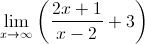#### Question 2 2. Using the squeeze theorem, what is z?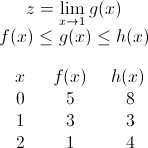#### Question 3 3. The floor function is graphically represented as a stepwise function. What is the limit of f(x) = floor(x) as x approaches 4?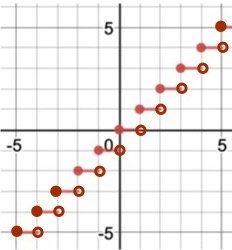#### Question 5 5. What is the limit of the function as it approaches x = -2 from the left side?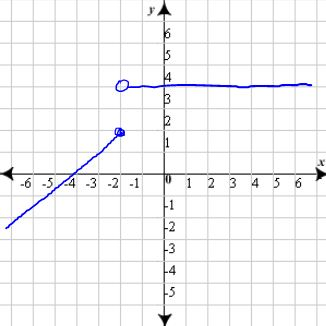### Page 2

#### Question 7 7. Using the given data, solve for y.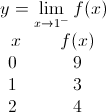#### Question 8 8. True or false: The following graph shows a continuous function.#### Question 9 9. The following limit is looking at which point in the function?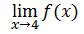#### Question 10 10. Use the graph to solve for z.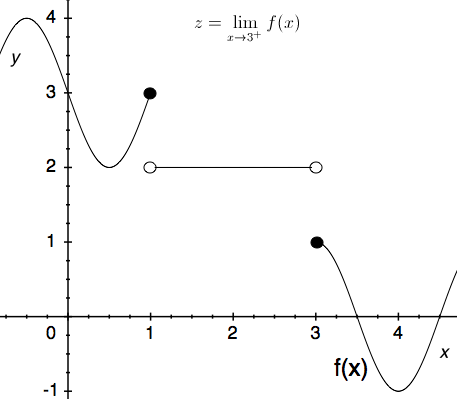### Page 3

#### Question 13 13. Calculate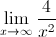#### Question 14 14. Using the squeeze theorem, what is z?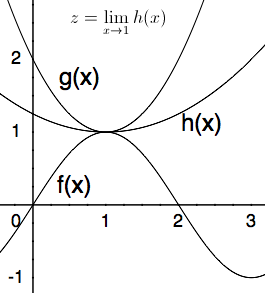#### Question 15 15. What is the limit of the function at x = 2?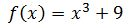### Page 4

#### Question 16 16. Calculate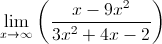#### Question 17 17. Using the squeeze theorem, what is z?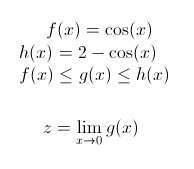#### Question 19 19. Evaluate the limit below.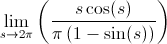### Page 5

#### Question 21 21. What is the limit?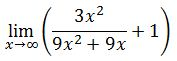#### Question 22 22. What is the limit as x approaches -2 of this function?#### Question 23 23. Using the squeeze theorem, what is z?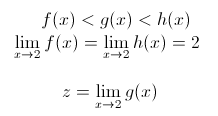#### Question 24 24. Calculate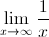### Page 6

#### Question 26 26. Use the graph to solve for z.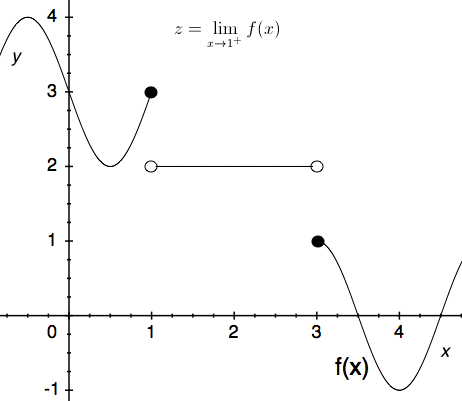#### Question 27 27. Using the given data, solve for y.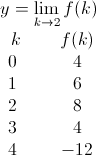#### Question 28 28. Use the graph to solve for z.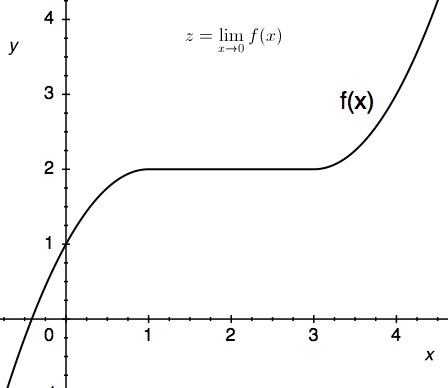#### Question 29 29. Find the limit.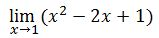#### Question 30 30. The ceiling function is graphically translated as a stepwise function. What is the limit of f(x) = ceil(x) as x approaches 4.5?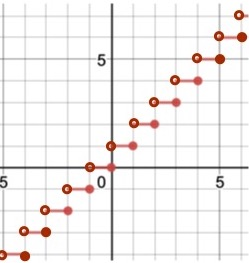#### Limits Chapter Exam Instructions

Choose your answers to the questions and click 'Next' to see the next set of questions. You can skip questions if you would like and come back to them later with the yellow "Go To First Skipped Question" button. When you have completed the practice exam, a green submit button will appear. Click it to see your results. Good luck!

Support